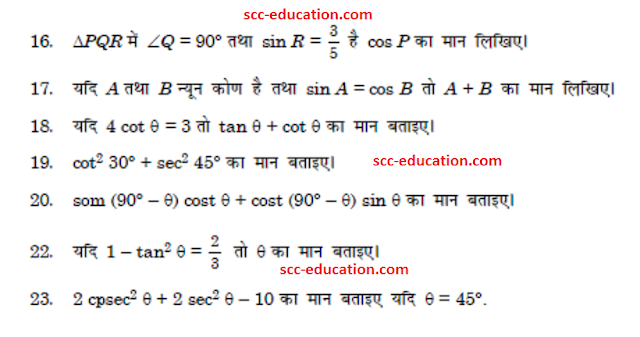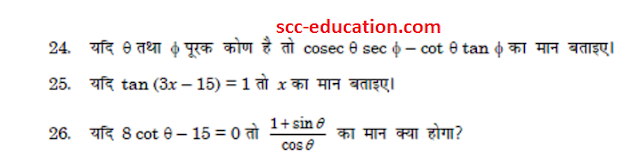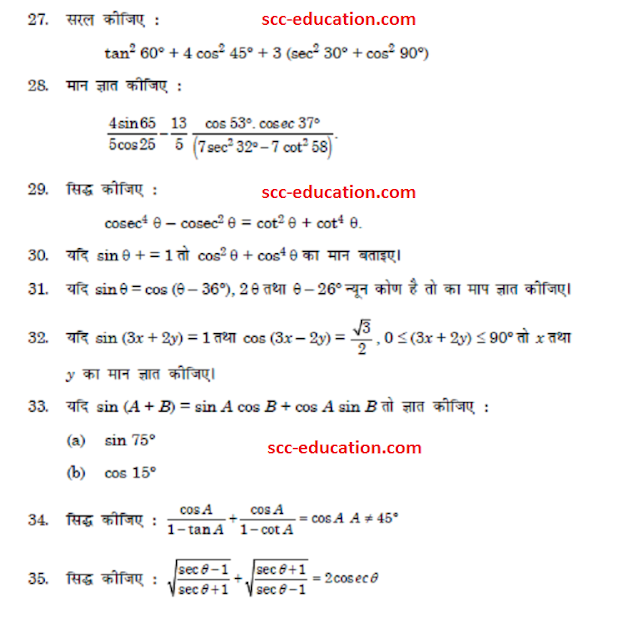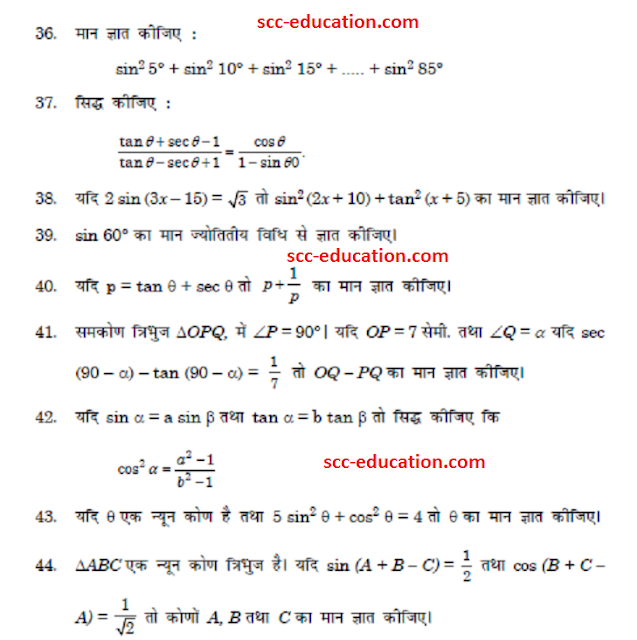# SCC Education

## Trigonometry in Hindi 2Basic-trigonometry-identities
graphing-linear-inequalities solving
Introduction-of-co-ordinate-geometry
probability
materials-metals-and-non-metals
Trignometry-solved-questions
surface-areas-and-volumes
sequence
linear-equations
Basic-trigonometry-identities

Introduction-of-co-ordinate-geometry

Probability

Materials-metals-and-non-metals

Trignometry-solved-questions

Surface-areas-and-volumes

Sequence

Linear-equations

Sample paper 1

Sample paper 2

Sample paper 3

Sample paper 4

Circle (Important Question)

Arithmetic Progression

circle (hindi)

Probability  (hindi)

### Polynomial in hindi